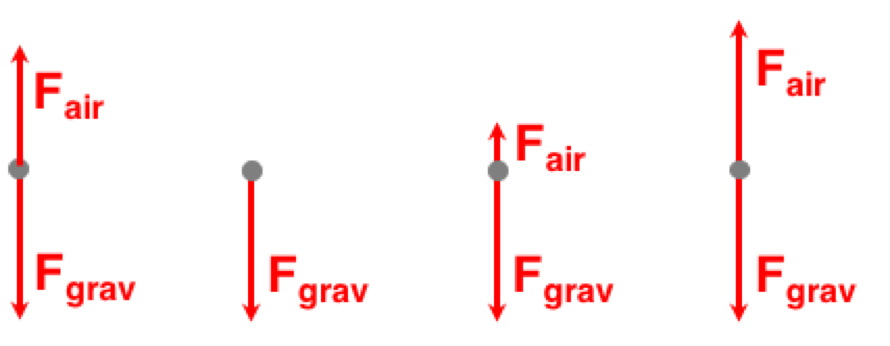Air Resistance and Skydiving - Questions

The Air Resistance and Skydiving Concept Builder is comprised of 12 Question Groups spread across three different activities. Each activity engages students in a slightly different task. The three activities are described as follows:

• Air Resistance: Question Groups 1-4 Identify the manner in which air density, object speed, and object cross-sectional area affect the air resistance force.
• Vector Diagrams: Question Groups 5-8 Identify the set of vector diagrams that show how the velocity, acceleration, air resistance force, and net force change over the course of a skydiver's fall.
• Velocity-Time Graph: Question Groups 9-12 Match free-body diagrams and information about the relative acceleration, net force, and air resistance to locations on a velocity-time graph representing a skydiver's motion.

The questions are shown below. Teachers are encouraged to view the questions in order to judge which activity is most appropriate for their classes.

The Physics Classroom grants teachers and other users the right to print these questions for private use. Users are also granted the right to copy the text and modify it for their own use. However, this document should not be uploaded to other servers for distribution to and/or display by others. The Physics Classroom website should remain the only website or server from which the document is distributed or displayed. We also provide a PDF that teachers can use under the same conditions. We have included a link to the PDF near the bottom of this page.

Air Resistance and Skydiving

Activity 1: Air Resistance
Question Group 1
Question 1
In what manner – increase or decrease – would the object speed, object cross-sectional area, and air density need to be changed in order to cause the air resistance to increase?

Question 2
In what manner – increase or decrease – would the object speed, object cross-sectional area, and air density need to be changed in order to cause the air resistance to increase?

Question 3
In what manner – increase or decrease – would the object speed, object cross-sectional area, and air density need to be changed in order to cause the air resistance to increase?

Question Group 2
Question 4
Consider the three spherical objects traveling through the same region of air. Radius and speed values are stated. Given that everything else is equal, rank them according to the amount of air resistance they encounter.Question 5
Consider the three spherical objects traveling through the same region of air. Radius and speed values are stated. Given that everything else is equal, rank them according to the amount of air resistance they encounter.Question 6
Consider the three spherical objects traveling through the same region of air. Radius and speed values are stated. Given that everything else is equal, rank them according to the amount of air resistance they encounter.Question Group 3
Question 7
Consider the three spherical objects traveling through the same region of air. Radius and speed values are stated. Given that everything else is equal, rank them according to the amount of air resistance they encounter.Question 8
Consider the three spherical objects traveling through the same region of air. Radius and speed values are stated. Given that everything else is equal, rank them according to the amount of air resistance they encounter.Question 9
Consider the three spherical objects traveling through the same region of air. Radius and speed values are stated. Given that everything else is equal, rank them according to the amount of air resistance they encounter.Question Group 4
Question 10
Consider the three spherical objects traveling through the same region of air. Radius and speed values are stated. Given that everything else is equal, rank them according to the amount of air resistance they encounter.Question 11
Consider the three spherical objects traveling through the same region of air. Radius and speed values are stated. Given that everything else is equal, rank them according to the amount of air resistance they encounter.Question 12
Consider the three spherical objects traveling through the same region of air. Radius and speed values are stated. Given that everything else is equal, rank them according to the amount of air resistance they encounter.Activity 2: Vector Diagrams
Question Group 5
Question 13
A skydiver is dropped out of an airplane at an altitude of 10000 feet. She reaches a terminal velocity 60 seconds later. Consider four positions during her fall.
A: Initial State (t = 0 seconds)
B: 15 Seconds After Drop
C:  45 seconds After Drop
D: 60 seconds After Drop
Toggle through the set of vector diagrams at the right to identify the relative magnitude of the velocity vector for each of these four positions. (Consider vertical motion only.)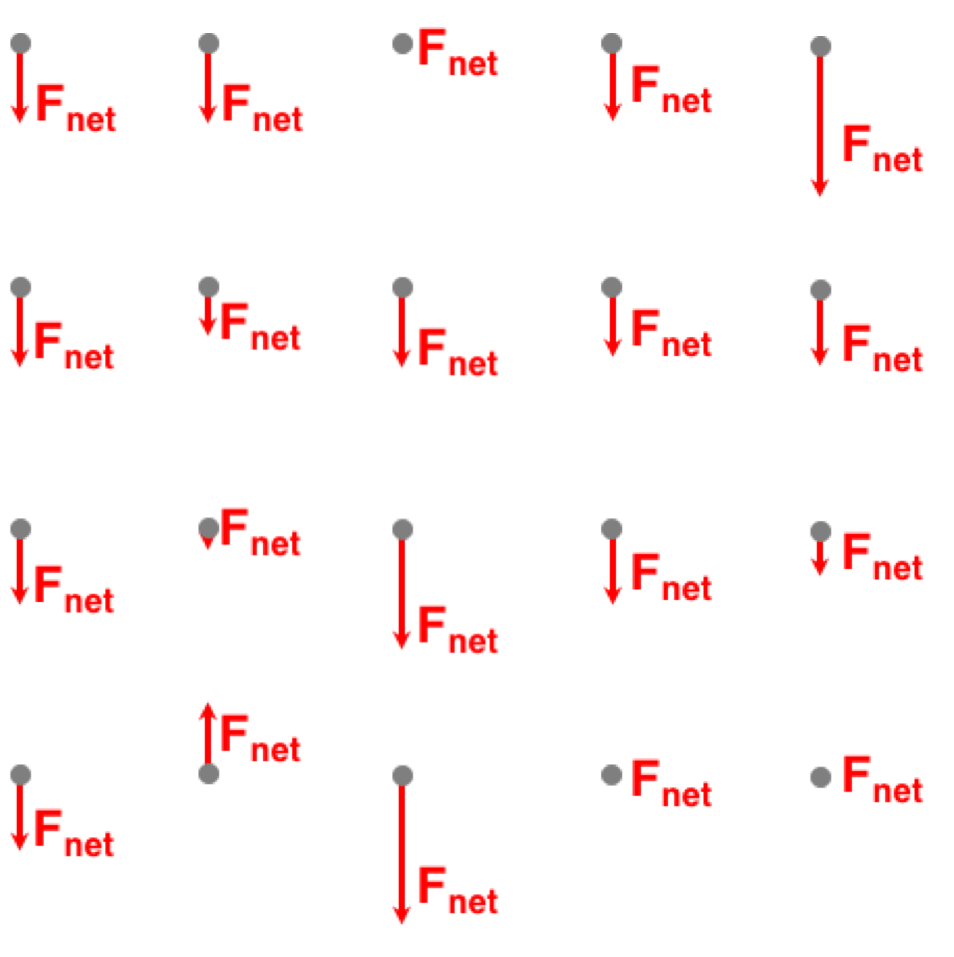Question Group 6
Question 14
A skydiver is dropped out of an airplane at an altitude of 10000 feet. He reaches a terminal velocity 40 seconds later. Consider four positions during her fall.
A: Initial State (t = 0 seconds)
B: 10 Seconds After Drop
C:  30 seconds After Drop
D: 40 seconds After Drop
Toggle through the set of vector diagrams at the right to identify the relative magnitude of the air resistance vector for each of these four positions. (Consider vertical motion only.)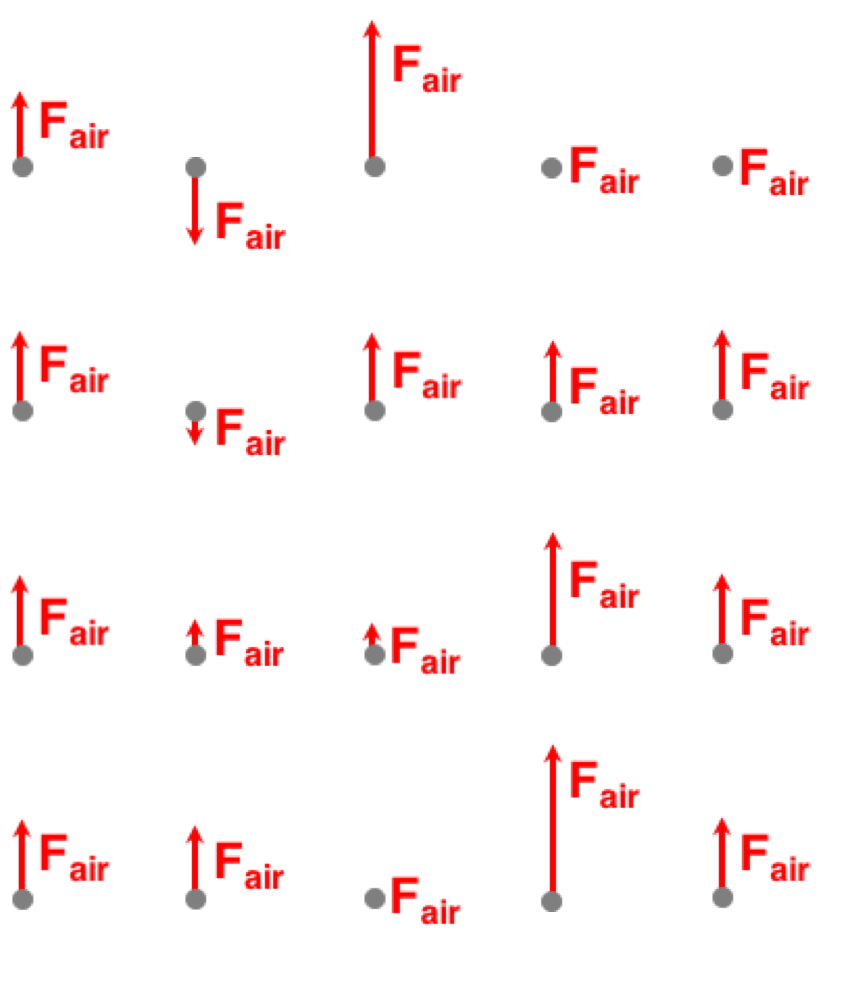Question Group 7
Question 15
A skydiver is dropped out of an airplane at an altitude of 10000 feet. She reaches a terminal velocity 60 seconds later. Consider four positions during her fall.
A: Initial State (t = 0 seconds)
B: 15 Seconds After Drop
C:  45 seconds After Drop
D: 60 seconds After Drop
Toggle through the set of vector diagrams at the right to identify the relative magnitude of the net force vector for each of these four positions. (Consider vertical motion only.)Question Group 8
Question 16
A skydiver is dropped out of an airplane at an altitude of 10000 feet. He reaches a terminal velocity 40 seconds later. Consider four positions during her fall.
A: Initial State (t = 0 seconds)
B: 10 Seconds After Drop
C:  30 seconds After Drop
D: 40 seconds After Drop
Toggle through the set of vector diagrams at the right to identify the relative magnitude of the acceleration vector for each of these four positions. (Consider vertical motion only.)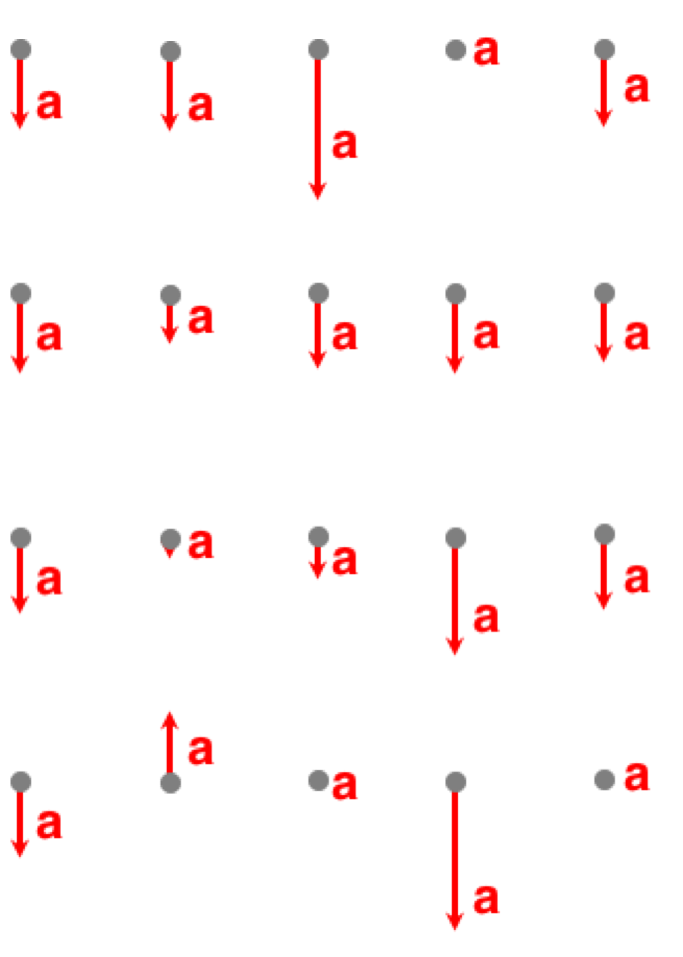Activity 3: Terminal Velocity
Question Group 9
Question 17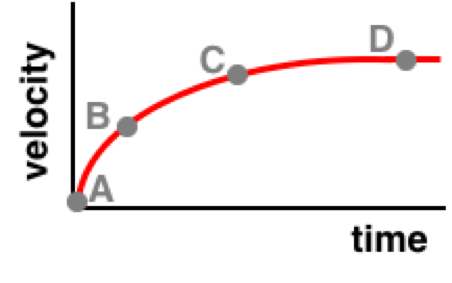A skydiver is dropped out of an airplane at an altitude of 10000 feet. The velocity-time graph at the right demonstrates how his velocity changes with time. (Note: down is defined as the + direction of velocity.) At what points during the skydive does he have the largest acceleration, a zero acceleration, and the smallest non-zero acceleration?

Acceleration is largest at ______.
Acceleration is zero at ______.
Acceleration is smallest (but not zero) at ______.

Question 18A skydiver is dropped out of an airplane at an altitude of 10000 feet. The velocity-time graph at the right demonstrates how his velocity changes with time. (Note: down is defined as the + direction of velocity.) At what points during the skydive does he have the largest acceleration, a zero acceleration, and the smallest non-zero acceleration?

Acceleration is zero at ______.
Acceleration is smallest (but not zero) at ______.
Acceleration is largest at ______.

Question 19A skydiver is dropped out of an airplane at an altitude of 10000 feet. The velocity-time graph at the right demonstrates how his velocity changes with time. (Note: down is defined as the + direction of velocity.) At what points during the skydive does he have the largest acceleration, a zero acceleration, and the smallest non-zero acceleration?

Acceleration is zero at ______.
Acceleration is largest at ______.
Acceleration is smallest (but not zero) at ______.

Question Group 10
Question 20A skydiver is dropped out of an airplane at an altitude of 10000 feet. The velocity-time graph at the right demonstrates how her velocity changes with time. (Note: down is defined as the + direction of velocity.) At what points during the skydive does she experience the greatest air resistance, zero air resistance, and the least (but non-zero) air resistance?

Air resistance is greatest at ______.
Air resistance is zero at ______.
Air resistance is least (but not zero) at ______.

Question 21A skydiver is dropped out of an airplane at an altitude of 10000 feet. The velocity-time graph at the right demonstrates how her velocity changes with time. (Note: down is defined as the + direction of velocity.) At what points during the skydive does she experience the greatest air resistance, zero air resistance, and the least (but non-zero) air resistance?

Air resistance is zero at ______.
Air resistance is least (but not zero) at ______.
Air resistance is greatest at ______.

Question 22A skydiver is dropped out of an airplane at an altitude of 10000 feet. The velocity-time graph at the right demonstrates how her velocity changes with time. (Note: down is defined as the + direction of velocity.) At what points during the skydive does she experience the greatest air resistance, zero air resistance, and the least (but non-zero) air resistance?

Air resistance is zero at ______.
Air resistance is greatest at ______.
Air resistance is least (but not zero) at ______.

Question Group 11
Question 23A skydiver is dropped out of an airplane at an altitude of 10000 feet. The velocity-time graph at the right demonstrates how her velocity changes with time. (Note: down is defined as the + direction of velocity.) At what points during the skydive does she have the experience the largest net force, a zero net force, and the smallest, non-zero net force?

Net force is largest at ______.
Net Force is zero at ______.
Net Force is smallest (but not zero) at ______.

Question 24A skydiver is dropped out of an airplane at an altitude of 10000 feet. The velocity-time graph at the right demonstrates how her velocity changes with time. (Note: down is defined as the + direction of velocity.) At what points during the skydive does she have the experience the largest net force, a zero net force, and the smallest, non-zero net force?

Net Force is zero at ______.
Net Force is smallest (but not zero) at ______.
Net force is largest at ______.

Question 25A skydiver is dropped out of an airplane at an altitude of 10000 feet. The velocity-time graph at the right demonstrates how her velocity changes with time. (Note: down is defined as the + direction of velocity.) At what points during the skydive does she have the experience the largest net force, a zero net force, and the smallest, non-zero net force?

Net Force is zero at ______.
Net force is largest at ______.
Net Force is smallest (but not zero) at ______.

Question Group 12
Question 26A skydiver is dropped out of an airplane at an altitude of 10000 feet. The velocity-time graph at the right demonstrates how his velocity changes with time. (Note: down is defined as the + direction of velocity.) Match each of the three free-body diagrams to one of the points on the graph.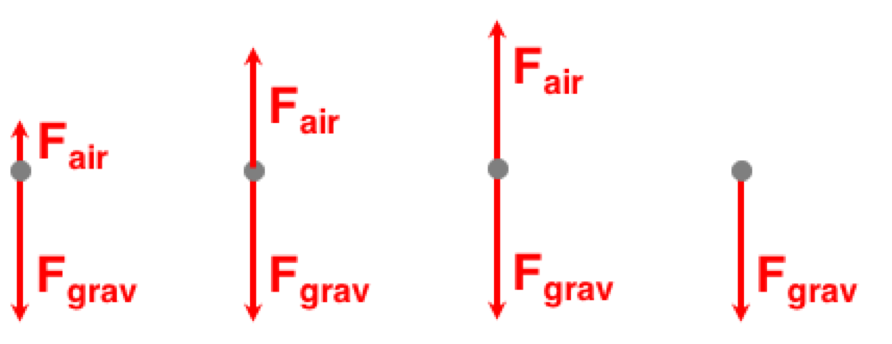Question 27A skydiver is dropped out of an airplane at an altitude of 10000 feet. The velocity-time graph at the right demonstrates how his velocity changes with time. (Note: down is defined as the + direction of velocity.) Match each of the three free-body diagrams to one of the points on the graph.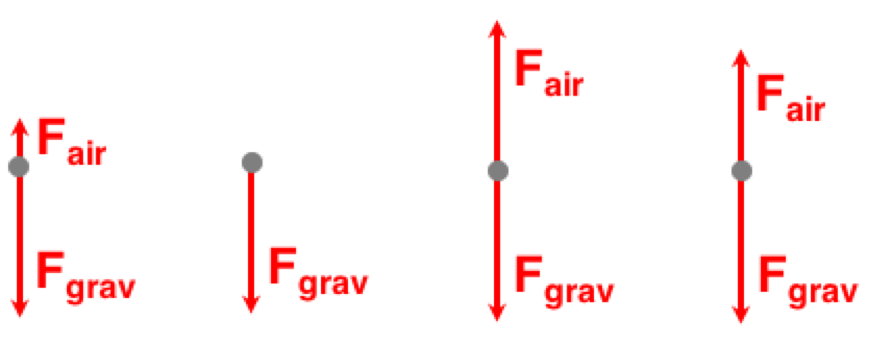Question 28A skydiver is dropped out of an airplane at an altitude of 10000 feet. The velocity-time graph at the right demonstrates how his velocity changes with time. (Note: down is defined as the + direction of velocity.) Match each of the three free-body diagrams to one of the points on the graph.